# Are You Trendy ? (Part 3)

Share

So you’ve made it to part 3 of Are You Trendy, well done.

In todays final post of Are You Trendy? we will take a break from the maths and discuss techniques and tools that Excel provides to assist us with Trend Analysis.

We will look at 2 techniques, one built-in to Excel called Charts and another which is a User Defined Function (UDF) I developed.

Both techniques have uses in analysis of trends.

Once again all examples below are found on the Examples Workbook.

## Excel Charts

Excel has a number of charting styles some of which have the ability to calculate and display Trend Lines.

The following tables shows which charts Do and Don’t support built-in trendlines.

 Supports Trendlines Doesn’t Support Trendlines Scatter (X Y) Pie Line Donut Area Radar Column Stock Bar Surface Bubble

For those charts that don’t support trendlines, this doesn’t stop us, as Excel users, from adding other series or using other techniques to add trendlines to the charts, it just that we have to do the hard work ourselves and not use Excels built-in skills.

For this exercise we will be using a Scatter (X Y) Chart but the techniques apply equally to all charts that support trendlines.

So lets go: First we need some data.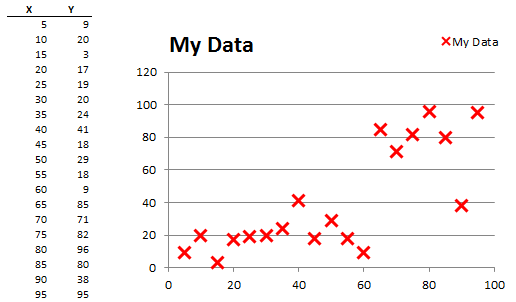Next add a scatter chart , Select the data and Insert Chart, X Y (Scatter Chart)

Adding a Trend Line to the chart is as simple as selecting the chart and right click on the Series you want to add the Trend Line to, see below.You can change the trendlines properties to make it stand out as per the above.

Once you add a Trendline a Format Trendline dialog will appear.This is the main window for setting Trendline properties and format options.

In this post I am not going to be dealing with the Tab Options of Line Color, Line Style, Shadow and Glow and Soft Edge options, as I am sure you can work out what they do and play with them at your leisure.

#### Trendline Properties

Once you have added a Trendline you can access the Trendlines properties at any time by:

Selecting the chart

Select the Trendline

Right Click and Format Trendline.

#### How Many Trendlines Can I Have ?

You can add a large number of Trendlines to each Chart Series in Excel by simply selecting the Right Clicking on the Chart Series and select Add Trendline.

Some of the regression types may not be available on second and subsequent regressions.

### Trend/Regression Type

The main section of the Format Trendline dialog is the Trend/Regression Type:

This section lists 5 trend types we are familiar with from the Are You Trendy? (Part 2).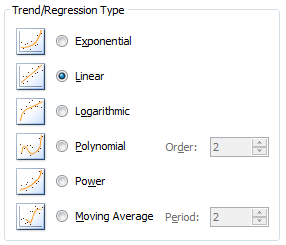For more details on the individual Tren Types refer to Are You Trendy (Part 2).

The default trend type is Linear ( Y = mX + c ).

You can select each type in turn and see the estimated trend.

#### Exponential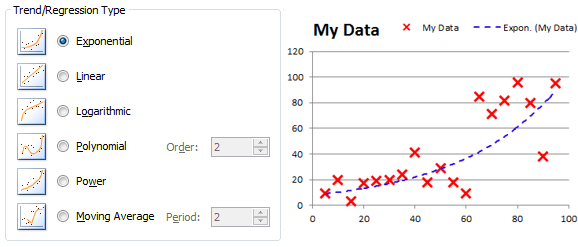#### Polynomial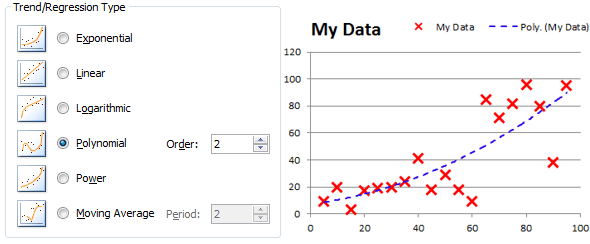This shows the Trendline for a Polynomial Estimate of Power = 2. Excel supports up to Power = 6.

#### Moving Average

Moving average isn’t a forecasting tool, but is more of a data manipulation tool, which is commonly used for elimination of short term trends or smoothing of the data source. Some industries use Moving Averages to look at buying and selling opportunities by comparing the current price against longer term trends.

It is possible to use moving average data for trend analysis, but this must be first done manually in Excel and then it can be used with the techniques discussed here.

As such we won’t be discussing its use here.

If you are interested in reading more about the use of moving averages have a look at  Moving Average.

### Trendline Name

The Trendline Name section by default shows you the Selected Trend Estimation type and your Data Series Name eg: Linear (My Data)

However you can type whatever Name you want by using the custom field:

eg: Huis Fruit Shop Sales Trend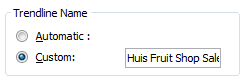### Forecast

The Excel Chart Trendline function allows us the option to project our Trendline a number of periods or X Values into the future or past.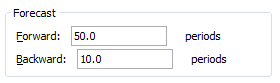The above will forecast our trend line 50 X axis values past the limits of our data and 10  X axis values prior to the limits of our data and is shown below.This is a great option to use for 2 reasons.

Firstly you can use this to see where the Trendline will cross the Y axis (X = 0) and is an important check to validate your selected Trendline.

You can see in the example above that the Trendline crosses the axis at -10. If this isn’t correct we can either try another estimation technique or Excel has a tool, which is discussed below, to deal with that.

Secondly, you can use it to look into the future by projecting the Trendline past your data limits.

### Misc Parameters

The final 3 parameters

#### Set Intercept

By default Excel Chart Trendline has used a Const value of True and hence calculates the natural intercept value for the data. If you want to over-ride this with a 0 intercept value Tick the box and select a value

Set Intercept : Not selected

Set Intercept : Selected Value of 10.0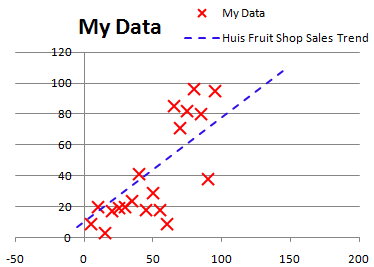I would always suggest using the Forecast backwards option first to see what the natural intercept is before using this option (Both charts above show the Trendline projected back 10 periods) but the second chart has a Y intercept set manually to 10.

#### Display Equation on Chart

The Display Equation on Chart options use is self explanatory, it Display the equation to the current Trendline on the current Chart:

The equation is based on the select Trend type.

The equation is also live, in that if you change and selected another Trend Type or the data changes the equation will update, similarly if you select to intercept the Y axis at 0 or another value.

By selecting the Text Box which has the equation the equations Font properties can be changed eg: Font Style, Size, Italic, Bold etc as well as background color.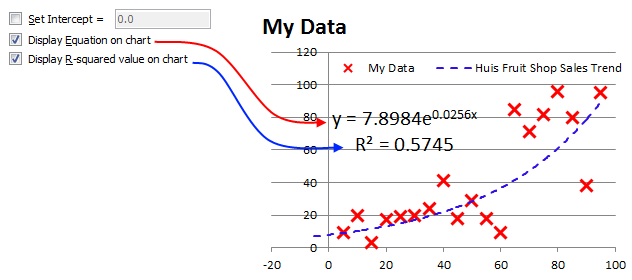#### Display R2 on Chart

This display the R2 value on the chart in the equation Text Box as shown above.

The R2 value is based on the select Trend type.

The R2 value is also live, in that if you change and selected another Trend Type or the data changes the R2 will update, similarly if you select to intercept the Y axis at 0 or another value.

By selecting the Text Box which has the equation the R2 the Font properties can be changed eg: Font Style, Size, Italic, Bold etc as well as background color.

### How Can We Look at Values Along a Trend Line?

In Are You Trendy? (Part 2) and in the section above we looked at a number of ways of establishing a Trendline.

So how can I predict values along a Trendline?

There are a few ways and we will discuss 2 of them.

1.       Write an equation in excel

2.       Use my Trendy User Defined function.

#### Write an Equation in Excel

In the previous section we had a chart shown below:

The equation for the line of best fit is Y=7.8984.e^(0.0256.X) which in Excel cell formula talk is =7.8984*EXP(0.0256*X Cell)

So we can setup a cell or range of cells in excel to show us either intermediate values or future values of X and the corresponding Y values.

The great thing about having an equation is that we can now use this to animate our chart.

I’m not talking about Avatar style animation, but simply adding a tool for the user to interactively select X values and the equation and chart will show us the new Y value. This even allows us to show future values past the end of our data because we are using the equation to our data.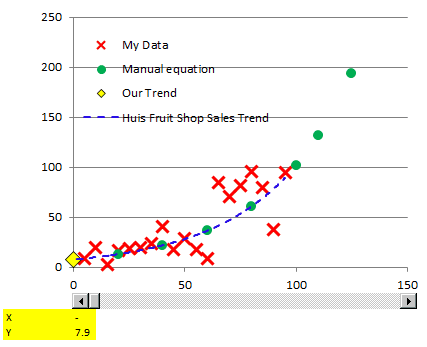### How do we do this?

Refer to Sample Workbook.

Firstly lets add a cell which will contain the X value

E52 = 10

Secondly add a cell which has our equation

E53:  =7.8984*EXP(0.0256*X Cell)

Third add a Scroll Bar Control from the Active X controls on the Developer toolbar and set a few properties

Minimum = 0

Maximum = 300

Large Change = 10

Fourth add a new series to our chart which will just have a single X and Y value

Series Name = “Our Trend”

X Series = E52

Y Series = E53

You can now interactively move the srcoll bar back and forth and the Chart will show you the new forecast value for our sales in Huis Fruit Shop.

The limitations with the above approach is that it is fixed to the equation you use for your Y value, which is based on your selected choice of Trendline type.

Can we link this technique to the equation from the Trendline ?

With Excel natively, No 🙁 .

## TrendY – A User Defined Function for the Analysis of Past, Intermediate & Future Trendline Values

In a post at Chandoo.org user Trevian3969 asked the question “How can I view intermediate values along a Charts trend line?”

The answer was the development of the UDF, TrendY (Trendy).

Trendy was developed to do exactly that, take the equation from a Charts Trendline and evaluate it to determine intermediate, past or future values based on a given X input.

Because the UDF is taking the equation for the trend line it can be used to return a Y value for any X values, prior to, later than or within the supplied or known X Range of the chart.

An Example of the use of Trendy is shown in the attached Workbook

Form:   = TrendY(X Value, [Chart No], [Series No], [Trendline No])

X Value: Is the X Value that you want to know the Y Value of the Trendline

Chart No: Chart No is optional and is the Chart No on the current sheet, Default or omitted = 1

Series No: Series No is optional and is the Series No on the chart, Default or omitted = 1. The series No is the number shown at the end of the Formula Bar when a series is selected

eg: =SERIES(“My Data”,Sheet1!\$B\$2:\$B\$41,Sheet1!\$C\$2:\$C\$41,1)

Trendline No: Trendline No is optional and is the Trendline No of the Trendline you wish to track. Default or omitted = 1.

[Option Parameters, Default = 1]

Eg:    = TrendY(20)  will place the value of X=20 into the Trendline Equation of the Trendline of Chart 1 Series 1 Trendline 1

= TrendY(40,1,3,2)  will place the value of X=20 into the Trendline Equation of the Trendline of Chart 1 Series 3 Trendline 2

=TrendY(B43,,3) will place the value of cell B43 into the Trendline Equation of the Trendline of Chart 1 Series 3 Trendline 1. Chart and Trendline both default to 1 as they are omitted.The function works with all the Trendline types and variants of those, except the Moving Average.

To use the Trendy UDF copy the following code to a VBA Module or alternatively import the Txt File as a new Module

Trendy Code Module

The code is also available in the Example Workbook with a fully working m0del.

If you don’t have the Trendlines equation shown it will do that for you and it ignores the R^2 function if shown.

The Trendlines equation box is actually where the function gets the equation from in the first place.

Then on a worksheet simply use then =Trendy(X Value) as described above.

Trendy has only been tested on Excel 2007 and 2010, use on other versions at your own risk.

### Limitations

The Trendy UDF is limited to use the available precision of the parameters, for each equation, as displayed in the Equation Text Box on the Chart.

In some cases especially when multiplying by powers of large numbers and especially in the Polynomial equations, there may be a large discrepancy between the calculated value by Trendy and the displayed value by the Trendline on the Chart. In these cases the Trendline is correct. This will show on the chart as the Plot Y value of Trendy not being on the Excel calculated Trendline.

Trendy has 2 constants at the top of the UDF that are used for the calculation of Intermediate Equations. These are equations that the user doesn’t see but are done at a much higher level of precision to avoid these errors. These constants are:

Const DataLabelNoFormat = “#,##0.0000”
Const PolynomialNoFormat = “#,##0.000000000000”

If you have rounding errors the easiest way to fix them is to increase the number of decimals by increasing the number of zeroes after the decimal in the appropriate line, remembering that Excel only carries 15 decimal places anyway and Trendy is already using 12 for Polynomial Trends already.

Trendy returns the displayed equations to 4 decimals after the intermediate calculations in any case.

Are You Trendy (Part 1)

Are You Trendy (Part 2)

All Examples from the Are You Trendy? Series in one Workbook

## Final

I hope you have enjoyed this 3 part series on Trend Analysis and Forecasting using Excel.

It has been put together to Introduce you to, and whet your appetite to, some of the functions, tools and techniques that you may not have had a lot of exposure to in the area of Trend Analysis and Forecasting using Excel.

I’d like to thank Chandoo for the opportunity to look after Chandoo.org for the past week and the opportunity to put the 4 posts up.

I will now hand Chandoo back the keys to the blog, hopefully without any dents.

Let me know what you thought of the series in the comments below:

Keep us informed on How you go with real data once you start applying some of these techniques.

### Hui…

ps: I still don’t know if Trevian3969 was happy with my response to his post as he never responded ?

### Get FREE Excel + Power BI Tips

Simple, fun and useful emails, once per week.

Learn & be awesome.

### Welcome to Chandoo.org

Thank you so much for visiting. My aim is to make you awesome in Excel & Power BI. I do this by sharing videos, tips, examples and downloads on this website. There are more than 1,000 pages with all things Excel, Power BI, Dashboards & VBA here. Go ahead and spend few minutes to be AWESOME.

Read my storyFREE Excel tips book

Chandoo is an awesome teacher
5/5

– Jason

Still on fence about Power BI? In this getting started guide, learn what is Power BI, how to get it and how to create your first report from scratch.

### 47 Responses to “Are You Trendy ? (Part 3)”

1. [...] This post was mentioned on Twitter by Stray__Cat, Excel Insider. Excel Insider said: Are You Trendy ? (Part 3): So you’ve made it to part 3 of Are You Trendy, well done. In todays final post of Are... http://bit.ly/gzdd3y [...]

2.Luke M says:

Excellent series Hui. I admit, it will probably take me awhile to fully digest all of it, as I don't have much previous experience working with trend analysis. But definitely a good reference for those times that I need to know.

3.Krok says:

Two thumbs up: I have just started to work in job that requires foceast skills and simple visualization based on excel, so this series helped me to (re)learn what I had forgot from school 😀

4.Jon Peltier says:

Good article, with a couple caveats.

1. You should encourage people NOT to use poly fits. There's no reason to believe the 6th order trendlines in your examples fit the data any better then a linear or at most 2nd order fit. A trendline type should be chosen based on an assumed underlying model of the charted behavior.

2. The data used in most of your data looks like it has two different populations, one up to X=60, and another (with an outlier) for X=65 and greater. If the X values are somehow sequential (i.e., date, series, number, production run number, etc.) you should be using some kind of control chart.

5.Tom Quist says:

Great post, Hui. Thanks for doing such a great job in place of Chandoo.

6.Tom Quist says:

@Jon - thanks for your thoughts on which trend type to use. So it's as simple as using your assumption of what underlying trend *should* be there? For seasonal data, I have used the previous year(s) to determine the trend. Is this an acceptable approach? If so - why or why not (anyone feel free to respond). Thanks!

7.Will says:

This has been on the best posts I've ever seen

8.Hui... says:

@Jon & Tom
Jon is correct in regards to selection of a trend estimator and I didn't spend enough time explaining that.

Nearly all data has some basis on which it is based and to use a simple example Electricity Pricing. You may have a fixed monthly connection charge and then be charged so much per kW for usage. So that should be linear from a fixed starting point. Variability comes in when comparing say electricity cost vs output of a factory. Even though the electricity will be directly related to the power consumed, the output may not and will have lots of other things impacting the output which may not necesarilly be connected to the power. like opening/closing stock level, holidays, plant breakdowns, special/rush orders to name a few.
It is rare to find real examples where as Jon said anything more than a Power 2 or 3 is required.

The important part is understanding your business drivers and then subsequently modeling it.

Jon - All the data was purely made up for demonstration purposes. I needed data which gave me room for Equation boxes and other things on screen and also needed enough variability to not just have everything as linear examples.
Control charts though are a management tool and not a trend analysis tool except for that they tell you your data isn't following historic trends and may have moved to another level as you pointed out.

9.Hui... says:

@Will, Luke, Tom, Krok & Jon
Thankyou for the appreciation.

10.Jon Peltier says:

Tom -

If you're looking at seasonal or cyclical data, then you may want to investigate a moving average. It smooths out the variability related to the cycles, and still shows irregularities.

For example, looking at web site hits, weekends are light, maybe 25% as much as weekdays. Looking at this data, all you notice is up and down on the weekly basis, and it's hard to see variation due, for example, to Thanksgiving, an American holiday that falls on a Thursday. But if you use a 7-point moving average, the peaks and valleys of weekdays and weekends are smoothed out, and you see the sizable dip for Thanksgiving, and again at Christmas and New Year's.

Then you might apply a trendline to your moving average data. For example, if it's a new web site and traffic is steadily increasing you may try a linear relationship.

11.Jon Peltier says:

The problem with overusing N-point polynomial regression is where the data is described by a mechanism that has its own theoretical trend. If you choose the best fit example of this particular trend, then the variation may represent measurement error or process variability. Since one important purpose of a trendline is for making predictions, you should stick to the best fit to the theoretical model, and not try to predict next week's values based on this month's variability.

In fact, the run chart approach usually assumes there is no trend, and that the variability stays the same from one period to the next, as long as the underlying process remains fixed. You can then predict themean value, as well as the probability that the actual value will fall within certain limits.

12.godzilla says:

@ Everyone! I am lovig this post and the commments! There is a really good discusssion going on here. My trand analysis is no where near the level you are discussing, however i would love to be.

Thanks guys!

13.Hedgehog says:

I have data with % on the y-axis and time on the x-axis. The curve is an exponential decay. I need to calculate the intercept of the trendline on the x-axis (ie y=0). At the moment I'm using INDEX and LOGEST to calculate my slope and intercept values and then algebraically solving for y=0, however I get a Log (0) in my equations, which of course doesn't work. Any ideas would be welcome.

14.Hui... says:

@Hedgehog
Sorry I can't answer that one.
Have a browse through http://newtonexcelbach.wordpress.com/
and maybe ask the same question there.

15. [...] Are You Trendy (Part 3) [...]

16.jay says:

Hello
great topic and I need some help. i need some help understanding the trendy formula.

I have the following set of range
x y
1 4
2 15
3 21
4 14
5 13
6 16
7 14
8 12
9 0 (unknown)
10 0(unknown)
11 0(unknown)
12 0 (unknown)

how i do the trendy for 12 (aka december)? basically y is the # of employees taht qualify for a prize, i need to know or get an idea by trendline how many there will be by 12 (month 12)...can someone pls help. i have data till month 8

thx u so much!

17.Hui... says:

@Jay
Assuming the posted Data started in A1 and 12 is in A13,
Select B10:B13
enter =TREND(B2:B9,A2:A9,A10:A13) Ctrl Shift enter
.
I would plot this and see how it looks

18.jay says:

hello Hui,

thxs for your prompt response. quick quick, I placed the trend formula in cell B10, do i need to make any of the cell references absolute?
i.e.
=TREND(\$C\$4:\$C\$11,\$B\$4:\$B\$11,\$B\$12:\$B\$15)
or do i make it
B10 = TREND(B2:B9,A2:A9,A10:A13) ---> drag it down to B13? (i ask bc on B13, the range for the formula goes into the blank cells i.e. =TREND(C7:C14,B7:B14,B15:B18)

19.Hui... says:

The instructions
"Select B10:B13
enter =TREND(B2:B9,A2:A9,A10:A13) Ctrl Shift enter "
.
Now type in or Paste =TREND(B2:B9,A2:A9,A10:A13)
Don't press Enter instead press Ctrl Shift Enter
.
Excel will put {=TREND(B2:B9,A2:A9,A10:A13) }
around the formula as it is an array entered formula
The Trend function does the rest

20.Jon Peltier says:

People don't get array formulas. Better to use this formula in B10, and fill it down:

=TREND(B\$2:B\$9,A\$2:A\$9,B10)

21.Hui... says:

Jon

=TREND(B\$2:B\$9,A\$2:A\$9,A10)
.
or I could have used
B10: =FORECAST(A10,\$B\$2:\$B\$9,\$A\$2:\$A\$9)
+ Copy down

22.jay says:

Hello Hui/Jon,

Thx u so much, both methods now work. Have a great day.

23.jay says:

Hey guys,

again thx u. final question. Based on this methodology, would it be safe to assume, hypothectically that, by month 12 (december), there would be approx 16.75 or 17 ppl that would qualify for the prize?
If i had to explain how month 12 gave 16.75, what would be the best way to approach this? thx you once again.

24.Hui... says:

Based on a linear regression of the first 9 months of data the estimate for the 12 months is...

25.Jon Peltier says:

And we should not blindly assume that regression points 1 through 9 provide the most valid predictions. If I regress points 2 through 9 (omitting the first point, which looks way lower than all the rest), I see my prediction drop from 12 in month 9 to 9.75 in month 12.

I don't know how to attach an image here, so I'll link to this:

http://peltiertech.com/images/2011-09/TwoTrends.png

Which prediction do you think is more valid? The one based on months 1 through 9, which shows a discontinuity between months 1 and 2, and between the data and the prediction? Or the one based on months 2 through 9, without the discontinuity in the data and between data and prediction?

26.Jon Peltier says:

Step 1: Look at the data
Step 2: Understand the data
Step 3: Determine how to make predictions based on the data.

27.Hui... says:

With reference to post 23 above, Hence the saying
"Lies, more lies and statistics"

28.Dave-O says:

"There are three kinds of lies: lies, damned lies, and statistics."-MT

29.Monica says:

Hi Hui and Chandoo,
I love the use of trendlines in my reports, however I can't seem to make my custom trendlines stick when I filter my pivots. Some filters work, others do not. Is it just not possible to do this? I hate to recreate the trendline each time, and the audience who uses the report will not be able to do this.

30.Hui... says:

@Monica
Trendlines (in a Chart I assume) are only made based on the charts data and as such if you filter data from the chart then the data is not available for use in the construction of the Trendline

Another way to do this would be to copy the original data,
Duplicate the series using the unfiltered copy of the data
Add a TrendLine to the new series,
Then make the series for the new Trendline have no color, so that you can only see the New Trendline and the original Filtered Data

31. [...] Part 3 – Trend Analysis & Forecasting using Charts & Macros [...]

32.jay196 says:

Take a chart of a year of stock prices for company AAA, with a 2nd and 3rd degree polynomial curve fitted. In general of course y(x) = sum[A(i)x^(i)], i=0,n
Thanks to your presentation above I finally found the equations and can show them in the chart.
Now we have AAA through ZZZ (or say 100-200 companies) and would rather extract the coefficients to a table.
How can this be done? Preferably to get the coefficients A(i), or a string with the equation that may be parsed.
I can do the calcs from the basic data, but prefer to just copy Excel's work! Thank you.

•Hui... says:

@Jay196
You can write a small VBA routine to extract the trendline coefficients or you can extract them directly from the data
VBA
```Sub Print_TL_Coefficients() For i = 1 To ActiveSheet.ChartObjects.Count   myTL = Worksheets(1).ChartObjects(i).Chart. _     SeriesCollection(1).Trendlines(1).DataLabel.Caption   Cells(i, 1).Value = myTL Next End Sub```

Directly from Source Data
Have a look at:

•jay196 says:

Thank you so much Hui!
Your code returns the equation in string form, from where it could be parsed. Using it as guidance I was able to dive into the object model (these things have dozens of undocumented properties/methods) and it looks like the coefficients are not exposed.
The link leads to a clever application of the linest function, which may be simplified by using it as an array function. That was what I used.
I see "experts" that know less than me, now I see there are Jedi Master levels way above.

33. [...] Are You Trendy (Part 3) [...]

34.Nagesh Prasad says:

Hi Hui,
A minor change is needed in the code to cater negative values of 'c' & 'b' in case of Linear and Logarithmic trend equations respectively.
Need to put check on 'x' instead of ' + ' for Logaritmic equation.
Need to remove -2 for below calculation in case of Linear equation.
c = Right(myTrend, Len(myTrend) - lngPtr - 2)

35.Leslie says:

Hi Hui;
I wonder if you can help me, Am trying to do some forecasting on student data, and the only data that I have to work with, are the following:

Their Predicted Points and their Actual Points
Plus students that have being accepted for a place.
The problem is, how can I use this data as the input values to do my forecasting?

Any ideas would be wonderful.

•Hui... says:

@Leslie
I would separate the data into two groups Accepted and Not Accepted
Then plot Actual vs Predicted and a a linear Trendline to each set of data
see if there are any trends in the data

You may also want to do a combined plot of both sets of data and apply a trendline

See what comes out of that

36.Leslie says:

Cool Hui;

I was going to try that one, as one of many algorithm I was going to developed.
I will just have to experiment and see.
Thanks.

•Hui... says:

I would also test the data by Class, Age, Sex and Teacher

37.Linar says:

Hi Hui
the excellent explanations. If ull have some more time pls keep posting of forecast skills in excel. BTW taking out this part can u advise any site or book about Forecasting in excel for beginers? I donot like a type of formulas such as mx+c. They drive me crazy - the better understanding is.coming with the practice so the examples will make it more clear. Thank u

•Hui... says:

@Linar

Have a browse at the Excel Bach website
https://newtonexcelbach.wordpress.com/
He does a fair bit with Forecasting

y = mx + c

is simply saying the Y Value is equal to the X Value x the Gradient (m) plus a constatnt (c)
Look at a Formula like y=2x+5
so if you have a Table of X Values say from 1 to 10
x y
0 5
1 7
2 9
3 11
4 13
5 15
6 17
7 19
8 21
9 23
10 25

You can see that for any Value of X you can calculate the Y value
This is great for forecasting values of say Sales, Costs etc

You will note two things

1. The Y value is the value of c (5) when x = 0
That is the minimum value of y is 5, assuming x can't be negative, when x =0
This is like the cost of running a business
Fixed Costs are incurred even if nothing is made

2. For each unit increase in x, y increases by m
Note above that going from 6 to 7 in x, y increases from 17 to 19 or m

This is the operating cost for example
if we make 5 units it will cost 15, if we make 6 units the extra unit costs 2 and so the total cost is now 17

3. If you now the Y Value you can back calculate the x Value
eg: if y=29
y=mx + c
29=2x + 5
24 = 2x
12 = x
So producing 12 units will result in a total cost of 29

The great thing about simple formulas like this is that they can be used for many many purposes and give precise answers, where as otherwise you may be using trial and error to derive a solution

38.Vikas says:

I have materials names with 6 years cost placed in front of them, I want to create a chart that can show the trend of cost year over year. Please help.

•Hui... says:

@Vikas

http://chandoo.org/forum/
Please attach a sample file to get a more targeted response

39.shabi says:

hi , thank you for your amazing page. please help me, I have problem at procedure for determining degree of best fitting polynomial.

### Get FREE Excel & Power-BI Newsletter

One email per week with Excel and Power BI goodness. Join 100,000+ others and get it free.